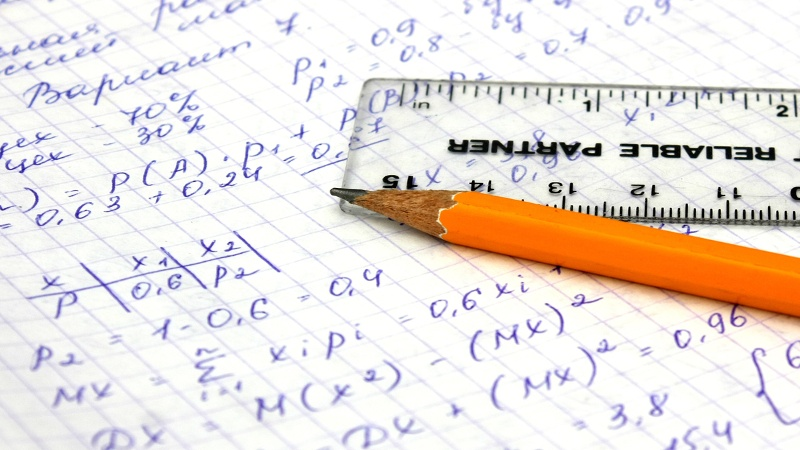ACT & SAT Prep and College Admissions BlogStudying for the SAT can seem like a daunting undertaking, but all you really have to do to master it is break it up and take it one section at a time. Today, we will be highlighting the Math section of the SAT.

Overall SAT-Math Format

There are actually two parts on the SAT that include math – a 25-minute non-calculator section and a 55-minute calculator section. Now, within both of these sections there are two problem types, the regular multiple choice with four answer choices as well as grid-in questions. In the first 25-minute non-calculator portion you will find 15 multiple choice questions and 5 grid-in questions. The 55-minute calculator portion will consist of 30 multiple choice questions and 8 grid-in questions.

Question Types

Now that you have a general overview of what to expect, let’s talk about what type of math problems to look out for. College Board has broken up the categories into the following: Heart of Algebra, Problem Solving and Data Analysis, Passport to Advanced Math, and Additional Topics in Math. Heart of Algebra focuses on algebra and linear equations while Problem Solving and Data Analysis measures your overall math literacy. The Passport to Advanced Math section, on the other hand, focuses on more complex math problems including complex equations. The final topic covered within the math section is going to be Additional Topics in Math, which addresses both geometric and trigonometric concepts.

Specific Topics

What topics should you specifically expect?

The Heart of Algebra questions will include:

• Linear Functions
• Linear Inequalities
• Graphical Representations
• Single Variable Equations
• Absolute Value

Under Problem Solving and Data Analysis you will see:

• Ratios
• Percentages
• Measurement Quantities
• Scatterplots and Graphs
• Mean, median, mode
• Standard deviation
• Categorical data and probabilities

The Passport to Advanced Math will include:

• Quadratic and Exponential Functions
• Rational Exponents
• Polynomial Expressions
• Nonlinear expressions
• Function Notation

Finally, the Additional Topics in Math includes:

• Volume Formulas
• Trigonometric Ratios and Pythagorean theorem
• Degrees and Radians
• Arc Lengths, Angle Measures, Chord Lengths, Areas of Sectors
• Congruence
• Sine and Cosine of Angles

Do not get overwhelmed at the range of topics you must master because, in reality, you already know many of these from math class in school. Your first step should be to take a practice test to diagnose what you need to work on and what you have already mastered. Once you take a practice test, look at the questions you got wrong and try to identify which section that was discussed above it falls into. Usually, it turns out that they will group together and all be the same underlying concept, and all you have to do is brush up on your volume formulas and suddenly you are getting 3 more questions correct.

Frequency of Question Types

Wondering what type of questions make up the majority of the math sections? Most agree that the highest percentage involves solving single variable equations, with a frequency of roughly 12%, and the next highest being defining and interpreting linear functions, at a frequency of roughly 11%. The least common skills that appear on the math section include function notation and solid geometry.

SAT Official Practice Tests

As you may be aware, they recently redesigned the SAT and due to this the number of official practice tests available are limited. However, there are still 8 available, and you should utilize each one to its full potential. Once you click on the following link, scroll down and practice away! The 8 Practice Tests include the questions, answers, as well as explanations to each question.

After taking the practice exams, you can compare your results to the average scores at your preferred universities/colleges. ThoughCo. provides a listing here.

Last Thoughts

As you go forth in beginning to prepare for the SAT, utilize all of your resources and ensure that you take practice exams under test conditions- including timing yourself. College Board also provides an app for your phone that allows you to instantly score your practice exams and it even has a “One Question a Day” feature that makes studying for the SAT a little more fun. Check out the app here! Good luck!